#### 期刊菜单

Rapid Detection Method of Tobacco Potato Virus X (PVX) and Study on the Inhibitory Effect of Resistance Inducer
DOI: 10.12677/HJAS.2020.109111, PDF, HTML, XML, 下载: 360  浏览: 1,122  科研立项经费支持

Abstract: The symptoms of tobacco seedlings after injection of PVX, the method of rapid detection of total RNA PVX infection of tobacco leaves, the diffusion of surrounding spots after treatment with antiviral resistance inducers, and the effect of friction inoculation resistance inducers were studied. The results showed that PVX could produce serious mosaic symptom in tobacco leaves after one week of injection; PVX was successfully expressed in tobacco leaves; RNA was extracted; the purity of PVX virus was detected by using the primers of virus; and the RNA OD260/280 = 1.955, c = 755.89 ng/μL was detected. The results showed that the infected leaves were caused by pure PVX. The virus spreading spot was smaller after 1 d treatment than that after 2 d treatment by UV irradiation and photo-taking. Under the stress of resistance inducer, the virus replication efficiency, movement and diffusion ability were all decreased, and the fluorescence spot of leaves treated by resistance inducer was enlarged, but the virus had not been transferred obviously. The results showed that PVX virus could be detected sensitively by extracting total RNA from tobacco leaves, which could be used as a rapid index to evaluate the infection of PVX virus. The resistance inducer (Bz) could inhibit the spread of potato X virus.

1. 引言

2. 材料与方法

2.1. 材料

2.1.1. 植物材料

2.1.2. 毒源材料

2.1.3. 生化试剂

RT-PCR反应试剂购自TaKaRa (大连宝生物工程有限公司)，通用型DNA纯化回收试剂盒(购自天根生化科技有限公司)，比施壮(重庆市优胜科技发展有限公司)。

2.1.4. PCR引物

Tabe 1. The primer of PVX CP and virus primers used commonly in laboratory

2.2. 方法

2.2.1. PVX纯病毒毒源的活化

1) 从−80℃冰箱中取出含PVX全长载体的农杆菌菌液5 μL。

2) 将5 μL菌液加入5 mL含有卡那霉素和链霉素YEP培养基中，225 rpm/min，30℃，培养12~16 h。

3) 从5 mL菌液中取1 mL加入浓度为20 μM的乙酰丁香酮(AS)5μL，加入到25 mL含有卡那霉素和链霉素YEP培养基中225 rpm/min，30℃培养12~16 h。

4) 从25 mL农杆菌菌液中取2 mL加入到50 mL离心管中，室温，5000 rpm/min离心15 min，弃上清。

5) 将沉淀用新配置的缓冲液重悬并调节OD600值至1.0~1.2，室温静置3 h。

$\begin{array}{l}{\text{MgCl}}_{\text{2}}\left(\text{1}\text{\hspace{0.17em}}\text{M}\right)\text{\hspace{0.17em}}\text{\hspace{0.17em}}\text{\hspace{0.17em}}\text{\hspace{0.17em}}\text{\hspace{0.17em}}\text{1}\text{\hspace{0.17em}}\text{mL}\\ \text{MES}\left(0.\text{5}\text{\hspace{0.17em}}\text{M}\right)\text{\hspace{0.17em}}\text{\hspace{0.17em}}\text{\hspace{0.17em}}\text{\hspace{0.17em}}\text{2}\text{\hspace{0.17em}}\text{mL}\\ \text{AS}\left(\text{1}00\text{\hspace{0.17em}}\text{mM}\right)\text{\hspace{0.17em}}\text{\hspace{0.17em}}\text{1}00\text{\hspace{0.17em}}\text{μL}\end{array}\right\}加灭菌水至\text{1}00\text{\hspace{0.17em}}\text{mL}$

6) 取培养至5~6叶期生长健壮的本氏烟，在叶片背面用针头打一小孔，用去掉针头的2.5 mL离心管吸取农杆菌悬浮液注射到叶片内。

2.2.2. 注射一周后观察症状并提取烟草中病毒总RNA

1) 称取0.1~0.2 g样品放入研钵。

2) 将500 μL RLT和50 μL Plantaid放入1.5 mL离心管中。

3) 用液氮研磨样品至细粉转入离心管内，立即剧烈震荡20 S，放入56℃水浴锅中3 min。

4) 将离心管从水浴锅中取出立即涡旋30 S，4℃离心机13,000 rpm离心10 min，将上清液转移至新的1.5 mL离心管中，加入0.5体积的无水乙醇。

5) 将混合液加入到吸附柱中(每次小于750 μL)，4℃离心机中13,000 rpm离心2 min，弃废液。

6) 在吸附柱中加入700 μL去蛋白液RW1，室温放置30 S，13,000 rpm离心30S，弃废液。

7) 在吸附柱中加入500 μL漂洗液RW，13,000 rpm离心30 S，弃废液并重复一次。

8) 将吸附柱放入收集管中，13,000 rpm离心2 min，弃废液。

9) 将吸附柱放入一新1.5 mL离心管中室温放置1 min，加入温度为70℃~80℃的RNase-free water 30~50 μL，13,000 rpm离心2 min。

10) 弃吸附柱，收集样品，并用2%琼脂糖凝胶检测提取结果，利用Goldview染色，用凝胶成像仪观察电泳结果。

2.2.3. RT-PCR

RT体系：

$\begin{array}{l}\text{5}×\text{Prime}\text{\hspace{0.17em}}\text{Suript}\text{\hspace{0.17em}}\text{Buffer}\text{\hspace{0.17em}}\text{\hspace{0.17em}}\text{\hspace{0.17em}}\text{\hspace{0.17em}}\text{\hspace{0.17em}}\text{\hspace{0.17em}}\text{\hspace{0.17em}}\text{ }\text{\hspace{0.17em}}\text{ }\text{ }\text{ }\text{ }\text{ }\text{ }\text{\hspace{0.17em}}\text{\hspace{0.17em}}\text{\hspace{0.17em}}\text{\hspace{0.17em}}\text{\hspace{0.17em}}\text{2}\text{\hspace{0.17em}}\text{μL}\\ \text{PrimeScript}\text{\hspace{0.17em}}\text{RT}\text{\hspace{0.17em}}\text{Enzyme}\text{\hspace{0.17em}}\text{\hspace{0.17em}}\text{\hspace{0.17em}}\text{\hspace{0.17em}}\text{ }\text{\hspace{0.17em}}\text{ }\text{\hspace{0.17em}}\text{ }\text{ }\text{\hspace{0.17em}}\text{\hspace{0.17em}}\text{\hspace{0.17em}}0.\text{5}\text{\hspace{0.17em}}\text{μL}\\ \text{Oligo}\text{\hspace{0.17em}}\text{dT}\text{\hspace{0.17em}}\text{Primer}\text{\hspace{0.17em}}\text{\hspace{0.17em}}\text{\hspace{0.17em}}\text{\hspace{0.17em}}\text{\hspace{0.17em}}\text{\hspace{0.17em}}\text{\hspace{0.17em}}\text{\hspace{0.17em}}\text{\hspace{0.17em}}\text{\hspace{0.17em}}\text{\hspace{0.17em}}\text{\hspace{0.17em}}\text{\hspace{0.17em}}\text{\hspace{0.17em}}\text{\hspace{0.17em}}\text{ }\text{\hspace{0.17em}}\text{\hspace{0.17em}}\text{ }\text{ }\text{ }\text{ }\text{\hspace{0.17em}}\text{\hspace{0.17em}}\text{\hspace{0.17em}}0.\text{5}\text{\hspace{0.17em}}\text{μL}\\ \text{Random}\text{\hspace{0.17em}}\text{6}\text{\hspace{0.17em}}\text{mers}\text{\hspace{0.17em}}\text{\hspace{0.17em}}\text{\hspace{0.17em}}\text{\hspace{0.17em}}\text{\hspace{0.17em}}\text{\hspace{0.17em}}\text{\hspace{0.17em}}\text{\hspace{0.17em}}\text{\hspace{0.17em}}\text{\hspace{0.17em}}\text{\hspace{0.17em}}\text{\hspace{0.17em}}\text{\hspace{0.17em}}\text{\hspace{0.17em}}\text{\hspace{0.17em}}\text{\hspace{0.17em}}\text{\hspace{0.17em}}\text{ }\text{ }\text{\hspace{0.17em}}\text{ }\text{ }\text{\hspace{0.17em}}\text{\hspace{0.17em}}\text{\hspace{0.17em}}0.\text{5}\text{\hspace{0.17em}}\text{μL}\\ \text{Total}\text{\hspace{0.17em}}\text{RNA}\text{\hspace{0.17em}}\text{\hspace{0.17em}}\text{\hspace{0.17em}}\text{\hspace{0.17em}}\text{\hspace{0.17em}}\text{\hspace{0.17em}}\text{\hspace{0.17em}}\text{\hspace{0.17em}}\text{\hspace{0.17em}}\text{\hspace{0.17em}}\text{\hspace{0.17em}}\text{\hspace{0.17em}}\text{\hspace{0.17em}}\text{\hspace{0.17em}}\text{\hspace{0.17em}}\text{\hspace{0.17em}}\text{\hspace{0.17em}}\text{\hspace{0.17em}}\text{\hspace{0.17em}}\text{\hspace{0.17em}}\text{\hspace{0.17em}}\text{\hspace{0.17em}}\text{ }\text{\hspace{0.17em}}\text{\hspace{0.17em}}\text{ }\text{\hspace{0.17em}}\text{\hspace{0.17em}}\text{\hspace{0.17em}}\text{\hspace{0.17em}}\text{2}.\text{5}\text{\hspace{0.17em}}\text{μL}\\ \text{RNase-free}\text{\hspace{0.17em}}{\text{ddH}}_{\text{2}}\text{O}\text{\hspace{0.17em}}\text{\hspace{0.17em}}\text{\hspace{0.17em}}\text{\hspace{0.17em}}\text{\hspace{0.17em}}\text{\hspace{0.17em}}\text{\hspace{0.17em}}\text{\hspace{0.17em}}\text{\hspace{0.17em}}\text{\hspace{0.17em}}\text{\hspace{0.17em}}\text{\hspace{0.17em}}\text{\hspace{0.17em}}\text{up}\text{\hspace{0.17em}}\text{to}\text{\hspace{0.17em}}\text{1}0\text{μL}\end{array}$

RT程序为：

$\text{37}℃\text{\hspace{0.17em}}\text{15}\text{\hspace{0.17em}}\text{min},\text{\hspace{0.17em}}\text{\hspace{0.17em}}\text{85}℃\text{\hspace{0.17em}}\text{2}0\text{S}$

PCR检测：

$\begin{array}{l}\text{1}0×\text{ExBuffer}\text{\hspace{0.17em}}\text{\hspace{0.17em}}\text{\hspace{0.17em}}\text{\hspace{0.17em}}\text{\hspace{0.17em}}\text{\hspace{0.17em}}\text{\hspace{0.17em}}\text{\hspace{0.17em}}\text{\hspace{0.17em}}\text{2}.\text{5}\text{\hspace{0.17em}}\text{μL}\\ {\text{MgCl}}_{\text{2}}\text{\hspace{0.17em}}\text{\hspace{0.17em}}\text{\hspace{0.17em}}\text{\hspace{0.17em}}\text{\hspace{0.17em}}\text{\hspace{0.17em}}\text{\hspace{0.17em}}\text{\hspace{0.17em}}\text{\hspace{0.17em}}\text{\hspace{0.17em}}\text{\hspace{0.17em}}\text{\hspace{0.17em}}\text{\hspace{0.17em}}\text{\hspace{0.17em}}\text{\hspace{0.17em}}\text{\hspace{0.17em}}\text{\hspace{0.17em}}\text{\hspace{0.17em}}\text{1}.\text{5}\text{\hspace{0.17em}}\text{μL}\\ \text{dNTP}\text{\hspace{0.17em}}\text{\hspace{0.17em}}\text{\hspace{0.17em}}\text{\hspace{0.17em}}\text{\hspace{0.17em}}\text{\hspace{0.17em}}\text{\hspace{0.17em}}\text{\hspace{0.17em}}\text{\hspace{0.17em}}\text{\hspace{0.17em}}\text{\hspace{0.17em}}\text{\hspace{0.17em}}\text{\hspace{0.17em}}\text{\hspace{0.17em}}\text{\hspace{0.17em}}\text{\hspace{0.17em}}\text{\hspace{0.17em}}\text{\hspace{0.17em}}\text{\hspace{0.17em}}0.\text{5}\text{\hspace{0.17em}}\text{μL}\\ 上游引物\text{\hspace{0.17em}}\text{\hspace{0.17em}}\text{\hspace{0.17em}}\text{\hspace{0.17em}}\text{\hspace{0.17em}}\text{\hspace{0.17em}}\text{\hspace{0.17em}}\text{\hspace{0.17em}}\text{\hspace{0.17em}}\text{\hspace{0.17em}}\text{\hspace{0.17em}}\text{\hspace{0.17em}}\text{\hspace{0.17em}}\text{\hspace{0.17em}}0.\text{5}\text{\hspace{0.17em}}\text{μL}\\ 下游引物\text{\hspace{0.17em}}\text{\hspace{0.17em}}\text{\hspace{0.17em}}\text{\hspace{0.17em}}\text{\hspace{0.17em}}\text{\hspace{0.17em}}\text{\hspace{0.17em}}\text{\hspace{0.17em}}\text{\hspace{0.17em}}\text{\hspace{0.17em}}\text{\hspace{0.17em}}\text{\hspace{0.17em}}\text{\hspace{0.17em}}\text{\hspace{0.17em}}0.\text{5}\text{\hspace{0.17em}}\text{μL}\\ \text{Ex}Taq酶\text{\hspace{0.17em}}\text{\hspace{0.17em}}\text{\hspace{0.17em}}\text{\hspace{0.17em}}\text{\hspace{0.17em}}\text{\hspace{0.17em}}\text{\hspace{0.17em}}\text{\hspace{0.17em}}\text{\hspace{0.17em}}\text{\hspace{0.17em}}\text{\hspace{0.17em}}\text{\hspace{0.17em}}\text{\hspace{0.17em}}\text{\hspace{0.17em}}\text{\hspace{0.17em}}0.\text{3}\text{\hspace{0.17em}}\text{μL}\\ \text{cDNA}\text{\hspace{0.17em}}\text{\hspace{0.17em}}\text{\hspace{0.17em}}\text{\hspace{0.17em}}\text{\hspace{0.17em}}\text{\hspace{0.17em}}\text{\hspace{0.17em}}\text{\hspace{0.17em}}\text{\hspace{0.17em}}\text{\hspace{0.17em}}\text{\hspace{0.17em}}\text{\hspace{0.17em}}\text{\hspace{0.17em}}\text{\hspace{0.17em}}\text{\hspace{0.17em}}\text{\hspace{0.17em}}\text{\hspace{0.17em}}\text{\hspace{0.17em}}\text{\hspace{0.17em}}\text{1}.\text{5}\text{\hspace{0.17em}}\text{μL}\\ {\text{ddH}}_{\text{2}}\text{O}\text{\hspace{0.17em}}\text{\hspace{0.17em}}\text{\hspace{0.17em}}\text{\hspace{0.17em}}\text{\hspace{0.17em}}\text{\hspace{0.17em}}\text{\hspace{0.17em}}\text{\hspace{0.17em}}\text{\hspace{0.17em}}\text{\hspace{0.17em}}\text{\hspace{0.17em}}\text{up}\text{\hspace{0.17em}}\text{to}\text{\hspace{0.17em}}\text{25}\text{\hspace{0.17em}}\text{μL}\end{array}$

$\begin{array}{l}\text{94}℃\text{\hspace{0.17em}}\text{\hspace{0.17em}}\text{\hspace{0.17em}}\text{3}\text{\hspace{0.17em}}\text{min}\\ \text{94}℃\text{\hspace{0.17em}}\text{\hspace{0.17em}}\text{\hspace{0.17em}}\text{45}\text{\hspace{0.17em}}\text{s}\\ \text{56}℃\text{\hspace{0.17em}}\text{\hspace{0.17em}}\text{\hspace{0.17em}}\text{45}\text{\hspace{0.17em}}\text{s}\\ \text{72}℃\text{\hspace{0.17em}}\text{\hspace{0.17em}}\text{\hspace{0.17em}}\text{9}0\text{\hspace{0.17em}}\text{s}\\ \text{72}℃\text{\hspace{0.17em}}\text{\hspace{0.17em}}\text{\hspace{0.17em}}延伸\text{1}0\text{\hspace{0.17em}}\text{min}\end{array}\right\}\text{3}0\text{\hspace{0.17em}}\text{cycles}$

2.2.4. 快速评价体系建立方法

1) 将含有的PVX-GFP载体的农杆菌菌液注射到本氏烟中。

2) 注射48 h之后利用共聚焦显微镜观察PVX-GFP在本氏烟中的表达情况。

3) 重新注射并在1 d后在注射叶片喷比施壮，3 d后利用UV灯观察注射点周围病毒扩散情况并拍照。

4) 另取一批苗子注射后喷清水作对照，同样利用UV灯观察并拍照，测定病毒扩增直径。

5) 利用半叶法将含有GFP标记病毒的叶片摩擦接种到健康烟苗中喷药并设置清水对照，观察喷药前后荧光斑点大小及数量。

3. 结果与分析

3.1. 注射PVX后烟苗的发病症状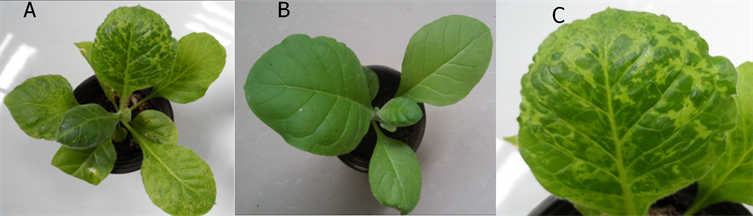注：A：注射一周后全株症状；B：为健康对照；C：注射一周后叶症状。

Figure 1. Mosaic symptom caused by PVX on cloud

3.2. PVX侵染烟草叶片总RNA的提取及PVX纯度检测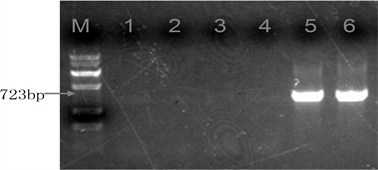注：1：TMV；2：CMV；3：TuMV；4：PVY；5：PVX；6阳性对照；M为Marker V。

Figure 2. Detection of several virus coat proteins by RT-PCR with specific primers

3.3. 在共聚焦显微镜下观察PVX-GFP的表达情况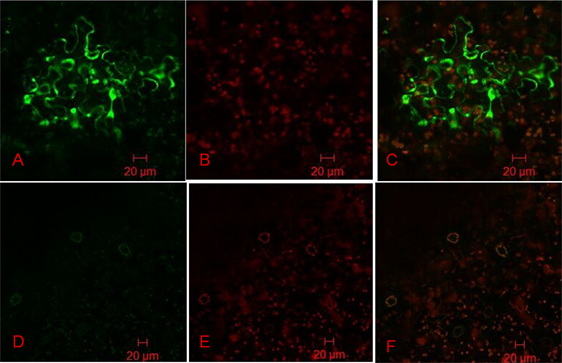注1：A、D：PVX-GFP处理和对照植物的荧光斑点；B、E：叶肉中叶绿素自发荧光；C、F：细胞中GFP及叶肉中叶绿素荧光叠加。注2：图中放大倍数为800倍。

Figure 3. Expression of PVX-GFP in tobacco leaves mediated by Agrobacterium tumefaciens

3.4. 用抗病毒抗性诱导物质处理后注射点周围病斑扩散情况

3.5. 摩擦法接种抗性诱导物质效果分析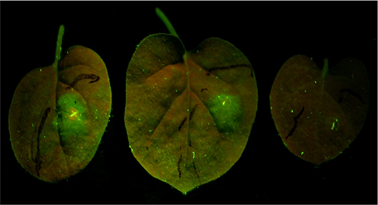Figure 4. Diffusion of fluorescent spots (from left to right in order of clear water control, Bishizhuang treatment, blank control)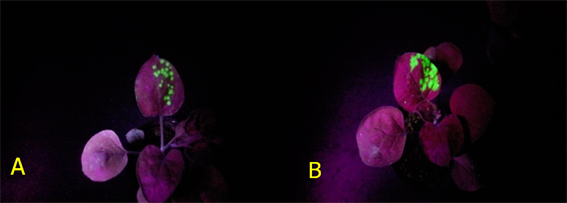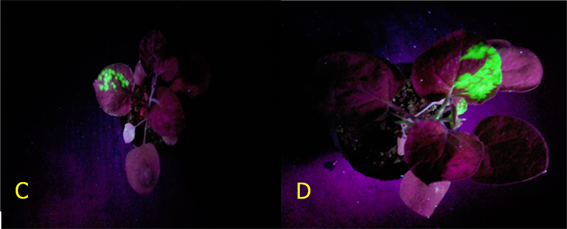注：A、C：比施壮处理2 d、3 d后荧光斑点；B、D：清水对照2 d、3 d后荧光斑点。

Figure 5. Virus fluorescent spots under UV light

4. 讨论

1) 将含有的PVX-GFP载体的农杆菌菌液注射到本氏烟中，在共聚焦显微镜下观察到，PVX-GFP在烟草细胞中具有较高的表达量，而对照组则没有荧光现象发生。

2) 烟草摩擦接种携带GFP的病毒，接种后利用比施壮处理，2 d后观察结果，比施壮处理的植株上病毒荧光斑点与清水处理相比，单斑荧光面积明显小，并且荧光强度明显弱。表明在抗性诱导物质胁迫下，病毒复制效率运动扩散能力都有所降低。植株根部随液流转移至新叶，而抗性诱导物质处理后的叶片荧光斑虽有所增长，但病毒尚未发生明显转移。

3) 植物抗性诱导物质室内筛选过程中，为了更好地明确植物病毒抑制的效果，通常都是用单一的纯病毒来作为研究对象。由于烟草病毒种类繁多，多种病毒复合侵染现象十分严重，导致病毒病症状表现更加复杂，在四川、云南、湖南等烟叶主产区均有相关报道。病毒的复合侵染会对植物症状产生较大影响，甚至能改变发病症状，使我们不能准确记录PVX纯病毒侵染烟苗后的症状，并且两种复合侵染还可能会在植物体中产生协同或拮抗作用，影响病毒在植物体中的传播。为了保证实验结果的可靠性，本实验利用实验室已保存的含有PVX-GFP瞬时表达载体的农杆菌菌液，注射到本氏烟中，利用多种实验室常见病毒特异性引物检测农杆菌注射后病毒纯度，结果表明，我们通过该方法获得了PVX病毒的纯毒源。因此该方法可有效防止病毒毒源在保存或后期接种过程中的交叉感染以及病毒在反复接种传代过程中的致病性变异，确保后续实验结果的准确性。

4) 本试验先利用共聚焦显微镜观察注射后烟苗确定PVX-GFP能够在本氏烟中大量表达，可知该毒源可应用到抗性诱导物质快速评价体系构建的实验中去。该体系能够快速直观地在实验室中观察到抗性诱导物质对病毒扩散过程中的抑制效果，我们利用一般寄主的汁液摩擦法进行实验，结果也再次验证了本方法室内筛选植物抗性诱导元素的可靠性。传统的抗性诱导物质评价系统使用半叶法 ，利用枯斑寄主接种观察，耗时长大约需要7~10天，并且要求必须是病毒的枯斑寄主，而本实验只需3~5天即可，且任何繁殖寄主均可以用来实验。

5) 本试验确立了一种实验室中新的评价体系思路，并为以后建立完善的抗性诱导物质药效评价体系奠定了基础。

  朱贤朝, 等. 主编. 中国烟草病害[M]. 北京: 中国农业出版社, 2002.  尚晓楠, 吴蓓蕾. 马铃薯X病毒荧光定量PCR检测体系的建立及应用[J]. 植物保护, 2016, 42(3): 165-169.  冯光惠, 杜虎平, 李夏隆, 亢福仁. 陕北地区马铃薯X病毒CP基因的RT-PCR检测及其序列分析[J]. 西北农林科技大学学报(自然科学版), 2014, 42(9): 91-96.  雷新云, 李怀方, 裘维蕃. 植物诱导抗性对病毒侵染的作用及诱导物质NS-83机制的探讨[J]. 中国农业科学, 1987, 20(4): 1-6.  Zhao, L., Hao, X.G. and Wu, Y.F. (2015) Inhibitory Effect of Polysaccharide Peptide (PSP) against Tobacco mosaic virus (TMV). International Journal of Biological Macromolecules, 75, 474-478. https://doi.org/10.1016/j.ijbiomac.2015.01.058